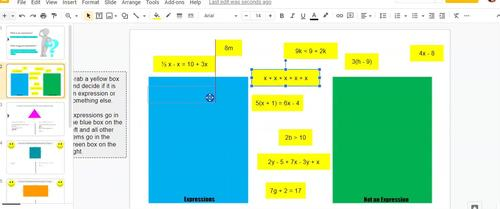# Simplifying Expressions Digital Activity;
7th - 9th
Subjects
Resource Type
Formats Included
• PDF
•Google Apps™
Pages
8 pages
Report this resource to TPTThe Teacher-Author indicated this resource includes assets from Google Workspace (e.g. docs, slides, etc.).

### Description

Students practice simplifying expressions through geometric application problems in this Google Slides activity. The idea is that many students have practiced this skill before but this lesson spotlights combining like terms and the distributive property while applying this knowledge to finding perimeter of simple figures such as equilateral triangles, squares and rectangles. The lesson comes with a video tutorial for simplifying expressions and a suggested homework activity from a Quizizz resource.

Here's what each slide contains:

• Slide 1: What is an Expression vs. What is Not an Expression
• Slide 2: Click and drag expressions and non-expressions to the correct box.
• Slide 3: An equilateral triangle with sides lengths labeled as expressions is used to find the perimeter in two ways. Students write about the different ways to work the problem.
• Slide 4: Students work a perimeter problem for a square two ways.
• Slide 5: Students work a perimeter problem for a rectangle two ways.
• Slide 6 & 7: Students work through distributing problems using a help video.
• Slide 8: Teacher Assignment Suggestion.
Total Pages
8 pages